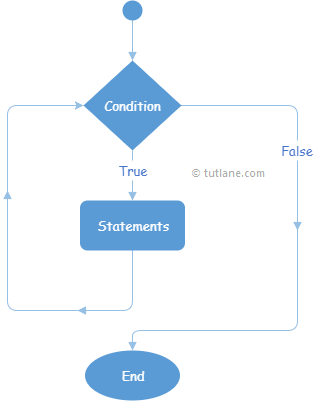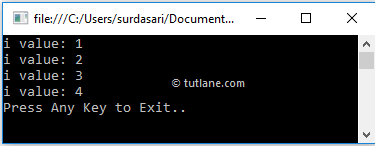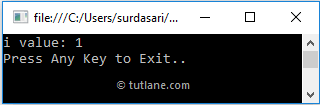# Visual Basic While Loop

In Visual Basic, While loop is useful to execute the block of statements as long as the specified condition is true.

In the previous section, we learned about for loop in visual basic with examples. Generally, the for loop is useful when we know how many times we need to execute the block of statements. In case we are unknown about the number of times to execute the block of statements, then `While` loop is the best solution.

## Visual Basic While Loop Syntax

Generally, we will use `While` keyword to create a while loop in Visual Basic applications. Following is the syntax of defining a while loop in Visual Basic programming language to execute the block of statements as long as the defined condition is true.

While boolean_expression
// Statements to Execute
End While

If you observe the above syntax, we used `While` keyword to define the while loop, and it contains a parameter called boolean_expression.

Here, if boolean_expression returns true, then the statements inside of the while loop will be executed. After executing the statements, again, the boolean_expression will be evaluated to execute the statements within the `While` loop.

In case the boolean_expression is evaluated to false, then the `While` loop stops the execution of statements, and the program will come out of the loop.

## Visual Basic While Loop Flow Chart

Following is the pictorial representation of `While` loop process flow in Visual Basic programming language.Now, we will see how to use `While` loop in Visual Basic programming language with examples.

## Visual Basic While Loop Example

Following is the example of using `While` loop in Visual Basic programming language to execute the block of statements based on our requirements.

Module Module1
Sub Main()
Dim i As Integer = 1
While i <= 4
Console.WriteLine("i value: {0}", i)
i += 1
End While
Console.WriteLine("Press Enter Key to Exit..")
End Sub
End Module

If you observe the above example, we are executing the statements within the `While` loop by checking the condition (i <= 4) and increasing the variable i (i++) value to 1 by using increment operator.

When we execute the above Visual Basic program, we will get the result as shown below.If you observe the above result, `While` loop has executed till it matches the defined condition (i <= 4), and the program comes out of the loop whenever the defined condition returns false.

## Visual Basic Nested While Loop

In Visual Basic, we can use one `While` loop within another `While` loop to implement the applications based on our requirements.

Following is the example of implementing the nested `While` loop in Visual Basic programming language.

Module Module1
Sub Main()
Dim i As Integer = 1
While i < 4
Console.WriteLine("i value: {0}", i)
i += 1
Dim j As Integer = 1
While j < 2
Console.WriteLine("j value: {0}", j)
j += 1
End While
End While
Console.WriteLine("Press Enter Key to Exit..")
End Sub
End Module

If you observe above example, we used one `While` loop within another `While` loop to achieve the nested while loop functionality in our application based on our requirements.

When we execute the above Visual Basic program, we will get the result as shown below.

i value: 1
j value: 1
i value: 2
j value: 1
i value: 3
j value: 1
Press Enter Key to Exit..

If you observe the above result, both `While` loops got executed and returned the result based on our requirements.

## Visual Basic While Loop with Exit Statement

In Visual Basic, we can exit or terminate the execution of `While` loop immediately by using `Exit` keyword.

Following is the example of using `Exit` keyword in `While` loop to terminate the execution of loop in Visual Basic programming language.

Module Module1
Sub Main()
Dim i As Integer = 1
While i < 4
Console.WriteLine("i value: {0}", i)
i += 1
If i = 2 Then Exit While
End While
Console.WriteLine("Press Enter Key to Exit..")
End Sub
End Module

If you observe the above example, whenever the variable (i) value become 2, we are terminating the loop using `Exit` statement.

When we execute the above Visual Basic program, we will get the result as shown below.This is how we can use the `Exit` statement with `While` loop to terminate the execution of the loop based on our requirements.